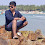## Problem:

We have triangle made of blocks. The topmost row has 1 block, the next row down has 2 blocks, the next row has 3 blocks, and so on. Compute recursively (no loops or multiplication) the total number of blocks in such a triangle with the given number of rows.

triangle(0) → 0
triangle(1) → 1
triangle(2) → 3## Solution:

public int triangle(int rows) {
if (rows == 0) return 0;
return rows + triangle(rows-1);
}

1.this is also the summation from i = 0 to i = n of i, so an alternative solution to the problem is simply n*(n+1)/2

2.public int triangle(int rows) {
return (rows ==0)? 0 : triangle(rows-1)+rows;
}

3.same result

public int triangle(int rows) {
if(rows == 0) {
return 0;
}

return rows + triangle(rows - 1);
}

4.return rows==0?0:rows+triangle(rows-1);